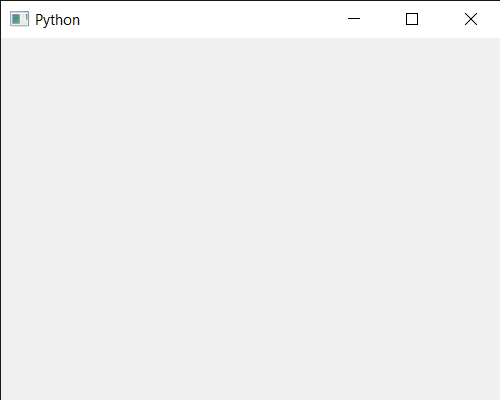Related Articles
PyQt5 QCalendarWidget – Setting Window Flags
• Last Updated : 17 Jun, 2020

In this article we will see how we can set the window flags of the QCalendarWidget. Window flags are a combination of a type (e.g. Qt::Dialog) and zero or more hints to the window system (e.g. Qt::FramelessWindowHint).If the calendar had type Qt::Widget or Qt::SubWindow and becomes a window (Qt::Window, Qt::Dialog, etc.), it is put at position (0, 0) on the desktop. If the widget is a window and becomes a Qt::Widget or Qt::SubWindow, it is put at position (0, 0) relative to its parent widget.

In order to do this we will use `setWindowFlags` method with the QCalendarWidget object.

Syntax : calendar.setWindowFlags(flag)

Argument : It takes window flag object as argument

Return : It return None

Below is the implementation

 `# importing libraries ` `from` `PyQt5.QtWidgets ``import` `*`  `from` `PyQt5 ``import` `QtCore, QtGui ` `from` `PyQt5.QtGui ``import` `*`  `from` `PyQt5.QtCore ``import` `*`  `import` `sys ` ` `  `# QCalendarWidget Class ` `class` `Calendar(QCalendarWidget): ` ` `  `    ``# constructor ` `    ``def` `__init__(``self``, parent ``=` `None``): ` `        ``super``(Calendar, ``self``).__init__(parent) ` ` `  ` `  ` `  `class` `Window(QMainWindow): ` ` `  `    ``def` `__init__(``self``): ` `        ``super``().__init__() ` ` `  `        ``# setting title ` `        ``self``.setWindowTitle(``"Python "``) ` ` `  `        ``# setting geometry ` `        ``self``.setGeometry(``100``, ``100``, ``500``, ``400``) ` ` `  `        ``# calling method ` `        ``self``.UiComponents() ` ` `  `        ``# showing all the widgets ` `        ``self``.show() ` ` `  ` `  `    ``# method for components ` `    ``def` `UiComponents(``self``): ` ` `  `        ``# creating a QCalendarWidget object ` `        ``# as Calendar class inherits QCalendarWidget ` `        ``self``.calendar ``=` `Calendar(``self``) ` ` `  `        ``# setting cursor ` `        ``self``.calendar.setCursor(Qt.PointingHandCursor) ` ` `  `        ``# setting size of the calendar ` `        ``self``.calendar.resize(``300``, ``240``) ` ` `  `        ``# move the calendar ` `        ``self``.calendar.move(``10``, ``10``) ` ` `  `        ``# setting windows flag ` `        ``self``.calendar.setWindowFlags(Qt.SplashScreen) ` ` `  ` `  `# create pyqt5 app ` `App ``=` `QApplication(sys.argv) ` ` `  `# create the instance of our Window ` `window ``=` `Window() ` ` `  `# start the app ` `sys.exit(App.``exec``()) `

Output :Attention geek! Strengthen your foundations with the Python Programming Foundation Course and learn the basics.

To begin with, your interview preparations Enhance your Data Structures concepts with the Python DS Course.

My Personal Notes arrow_drop_up
Recommended Articles
Page :